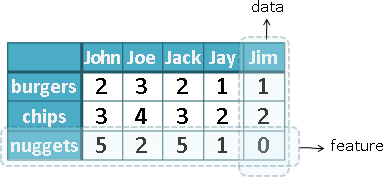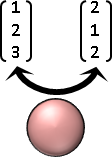Data

DataGraphp = {{2,3,5},{3,4,2},{2,3,5},{1,2,1},{1,2,0}}; l = {{p[],p[]},{p[],p[]},{p[],p[]},{p[],p[]},{p[],p[]}}; Graphics3D[{Black, Specularity[White, 10], Sphere[p, 0.3], Thickness[0.03], CapForm["Butt"], RGBColor[255,192,0], Opacity[0.9], Line[l]}, PlotRange -> {{-1,6},{-1,6},{-1,6}}, Boxed -> False]

Measure```一、長度不會是負數。p(A) ≥ 0

```

```L₀ Norm：非零的數量。
L₁ Norm：先轉正數、再相加。
L₂ Norm：先平方和、再平方根。
L∞ Norm：最大值。
``````一個數值的長度：用絕對值計算長度。

最經典的是平方長度：先平方和、再平方根。

```

```一個集合的長度：「Set Rank」

``````一、距離不會是負數。d(A,B) ≥ 0

d(A,B) + d(B,C) ≥ d(A,C)
或者說，三個東西融合成兩個東西，其距離只會累加或短少。
d(A,C) ≤ d(A,B) + d(B,C)
```

```Euclidean Distance（L₂）：直線距離。
Taxicab Distance（L₁）：垂直、水平移動的距離。
Hamming Distance（L₀）：相對應維度，數值相異的維度個數。
``````兩個數值的距離：用減法與絕對值計算距離。

重新表示訊號，再用數學公式計算距離。

```

UVa 10508 11085 ICPC 5132

```兩個向量的距離：「Cosine Distance」

```

```二、恰是零的東西，長度等於零。p(A) = 0 when A = 0

```
```二、兩個相同的東西，距離等於零。d(A,B) = 0 when A = B

```